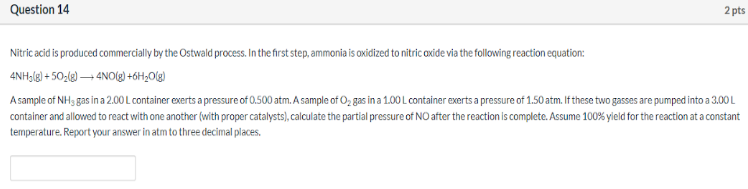# Nitric acid is produced commercially by the Ostwald process. In the first step, ammonia is oxidized to nitric oxide via the following reaction equation: 4NH3(g) + 5O2(g) → 4NO(g) + 6H2O(g) A sample of NH3 gas in a 2.00 L container exerts a pressure of 0.500 atm. A sample of O2 gas in a 1.00 L container exerts a pressure of 1.50 atm. If these two gasses are pumped into a 3.00 L container and allowed to react with one another (with proper catalyst), calculate the partial pressure of NO after the reaction is complete. Assume 100% yield for the reaction at a constant temperature. Report your answer in atm to three decimal places.